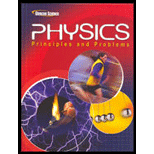# The reason that an electric range and an electric hot-water heater would be connected to a 240 -V circuit rather than to a 120 -V circuit.### Glencoe Physics: Principles and Pr...

1st Edition
Paul W. Zitzewitz
Publisher: Glencoe/McGraw-Hill
ISBN: 9780078807213### Glencoe Physics: Principles and Pr...

1st Edition
Paul W. Zitzewitz
Publisher: Glencoe/McGraw-Hill
ISBN: 9780078807213

#### Solutions

Chapter
Section
Chapter 22.2, Problem 38SSC
To determine

## The reason that an electric range and an electric hot-water heater would be connected to a 240-V circuit rather than to a 120-V circuit.

Expert Solution

To have less thermal energy loss,

### Explanation of Solution

Introduction:

The electric power (P) is given by,

P=IV

Where, I is the current and V is the potential difference.

And, the thermal energy (E) during time t is given by,

E=Pt=I2tR

Where,

P=Power dissipatedI= CurrentR=Resistance

Considering the above mentioned formula for the power, for the same power if the voltage is more, then the current will be less.

Then, considering the formula for the thermal energy loss, if the current through the circuit is less, the thermal energy loss will also be less.

Conclusion:

Hence, to have the less loss due to thermal energy, an electric range and an electric hot-water heater would be connected to a 240-V circuit rather than to a 120-V circuit.

### Have a homework question?

Subscribe to bartleby learn! Ask subject matter experts 30 homework questions each month. Plus, you’ll have access to millions of step-by-step textbook answers!